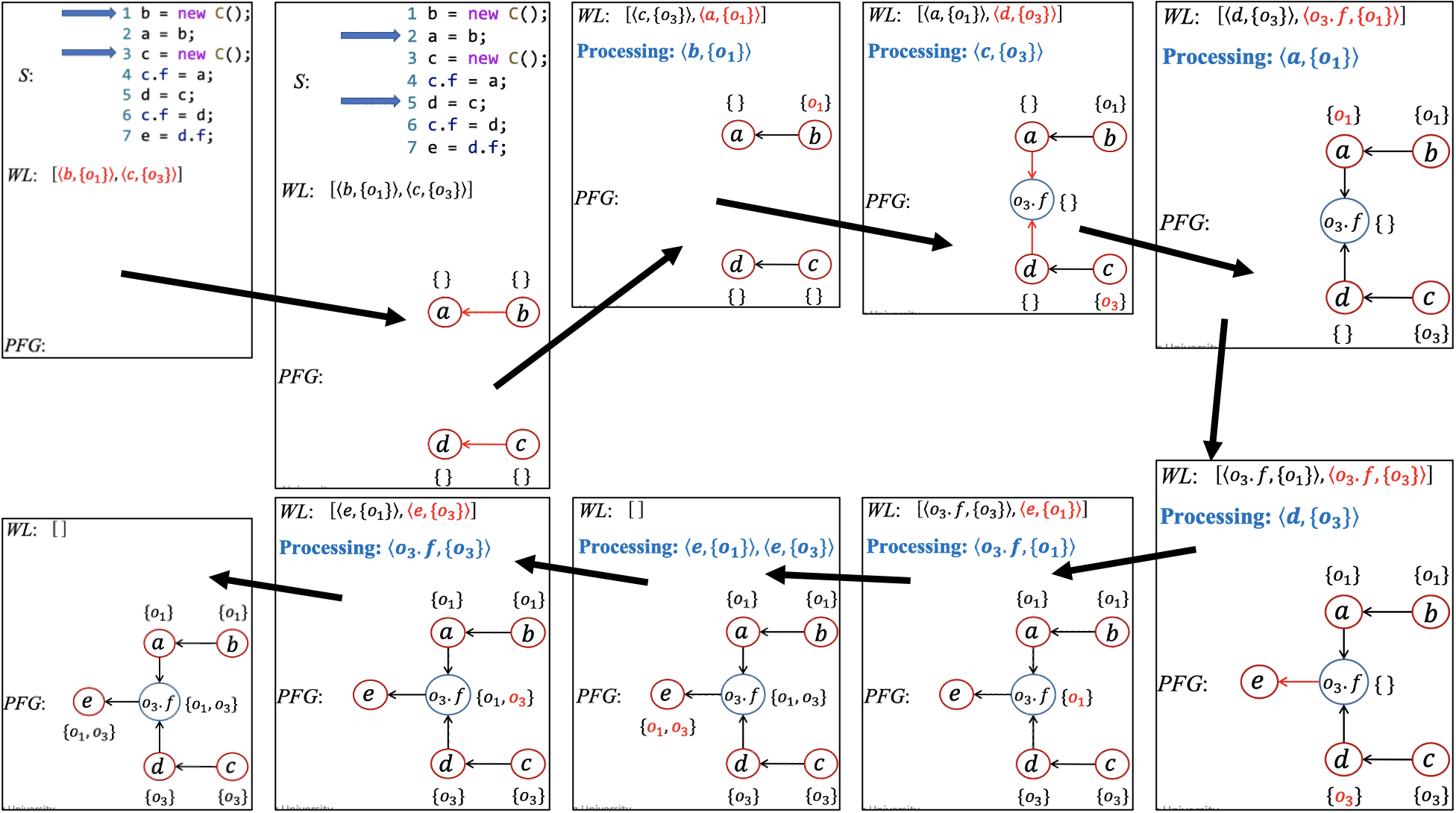## 指针分析规则

• Variables: $x, y \in V$
• Fields: $f, g \in F$
• Objects: $o_{i}, o_{j} \in O$
• Instance fields: $o_{i}.f, o_{j}.g \in O \times F$
• Pointers: $\text{Pointer} = V \cup (O \times F)$
• Points-to relations: $pt: \text{Pointer} \rightarrow P(O)$

New i: x = new T() $\frac{}{o_{i} \in pt(x)}$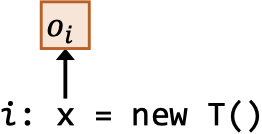Assign x = y $\frac{o_{i} \in pt(y)}{o_{i} \in pt(x)}$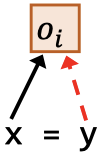Store x.f = y $\frac{o_{i} \in pt(x),\ o_{j} \in pt(y)}{o_{j} \in pt(o_{i}.f)}$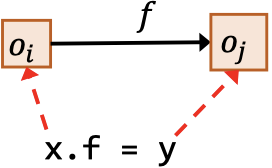Load y = x.f $\frac{o_{i} \in pt(x),\ o_{j} \in pt(o_{i}.f)}{o_{j} \in pt(y)}$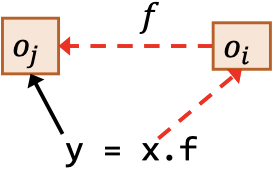## 指针分析实现

PFG的节点就是指针（$\text{Pointer} = V \cup (O \times F)$），代表变量或对象的域成员；对于边（$\text{Pointer} \times \text{Pointer}$）来说，一个$x \rightarrow y$的边表示指针$x$指向的对象可能（may）会流动到指针$y$。PFG的边需要根据中的程序语句和前面介绍的处理规则来添加：

New i: x = new T() $\frac{}{o_{i} \in pt(x)}$ N/A
Assign x = y $\frac{o_{i} \in pt(y)}{o_{i} \in pt(x)}$ $x \leftarrow y$
Store x.f = y $\frac{o_{i} \in pt(x),\ o_{j} \in pt(y)}{o_{j} \in pt(o_{i}.f)}$ $o_{i}.f \leftarrow y$
Load y = x.f $\frac{o_{i} \in pt(x),\ o_{j} \in pt(o_{i}.f)}{o_{j} \in pt(y)}$ $y \leftarrow o_{i}.f$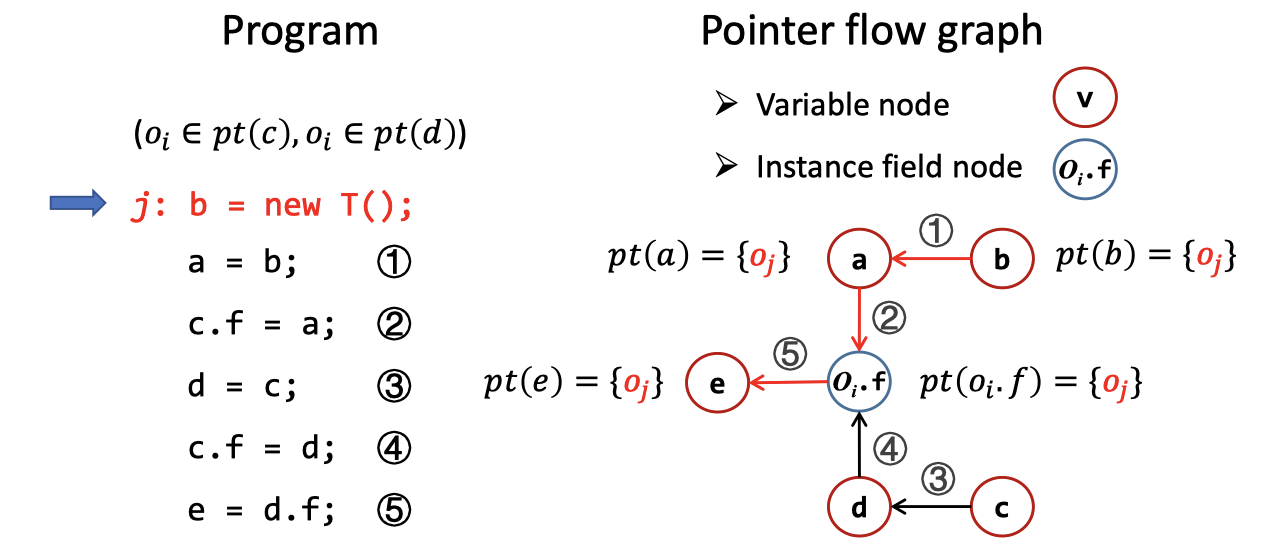## 指针分析算法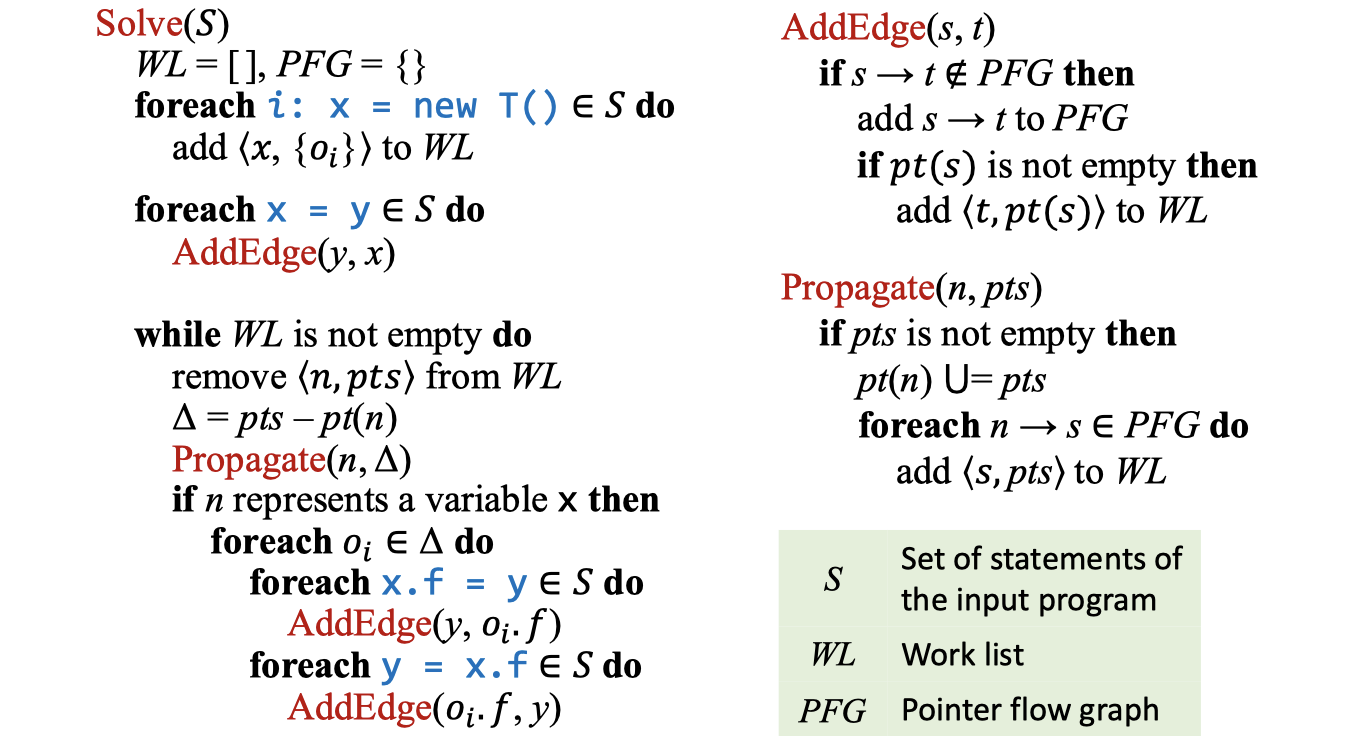Worklist包含了待处理的指向信息：$WL \subseteq \langle \text{Pointer}, P(O) \rangle ^\ast$。WL的每一项$\langle n, pts \rangle$都是指针$n$和指向对象集合$pts$构成的对，这些信息需要被传播到$n$的现有指向集合$pt(n)$中。

$$\Delta = pts - pt(n) \\ Propagate(n,\Delta)$$